Previous: Rotating Objects  Up: Selecting and Editing Objects  Next: Shearing Objects

# Scaling Objects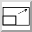Selected objects can be scaled either by clicking on the scale button or by selecting Transform->Scale (Ctrl-Z). This will open up a dialog box in which you can specify the scale factor. (There is a choice of scaling just the x dimension, just the y dimension or both dimensions.)

Notes:

• Individual objects will be scaled relative to the top left corner of the object's bounding box.

• Objects within a group will be scaled relative to the top left corner of the group's bounding box.

• Scaling a text-path will scale the path and the text will adjust to follow the transformed path. Note that the text itself will not be scaled.

• Scaling a text area and path and then combining them to form a text-path is not the same as first combining and then scaling.

To illustrate this, in Figure 8.20(a) there are three objects selected. The selection is then scaled by a factor of 2. The result is shown in Figure 8.20(b).

In Figure 8.21, the three objects in Figure 8.20 were first grouped (Figure 8.21(a)) and then scaled by a factor of 2 (Figure 8.21(b)).

In Figure 8.22, the path and text area in Figure 8.22(a) are combined into a text-path, shown in Figure 8.22(b). This text-path is then scaled by a factor of 2 resulting in Figure 8.22(c). Note that this is different from first scaling the original path and text area, shown in Figure 8.22(d), and then combining them to form a text-path, shown in Figure 8.22(e).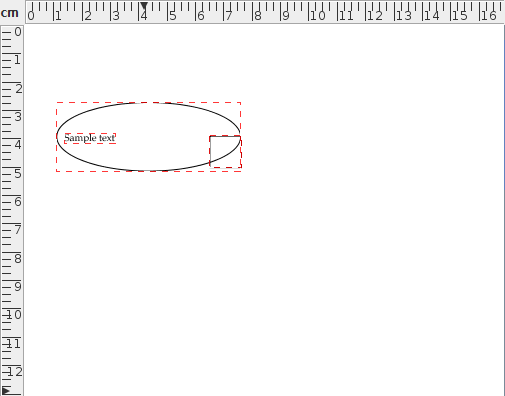(a)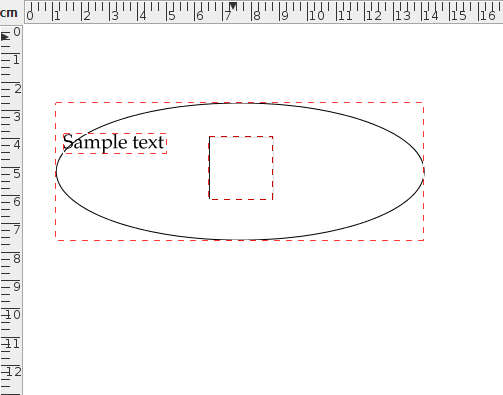(b)
Figure 8.20: Three selected objects scaled by a factor of 2: (a) before, (b) after.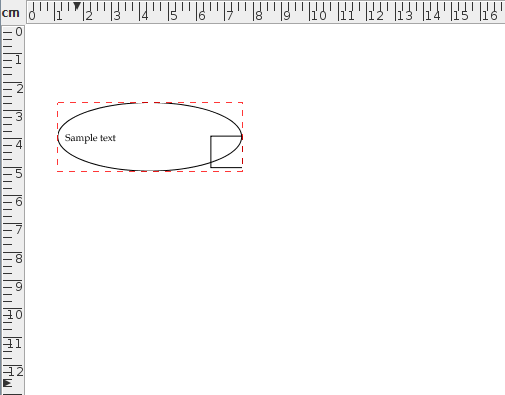(a)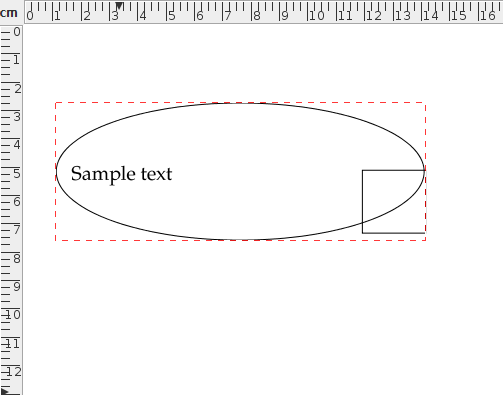(b)
Figure 8.21: A group consisting of three objects scaled by a factor of 2: (a) before, (b) after.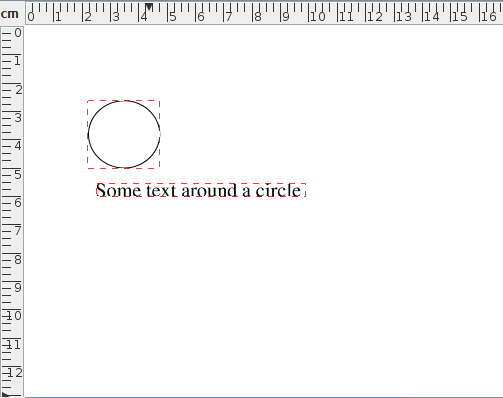(a)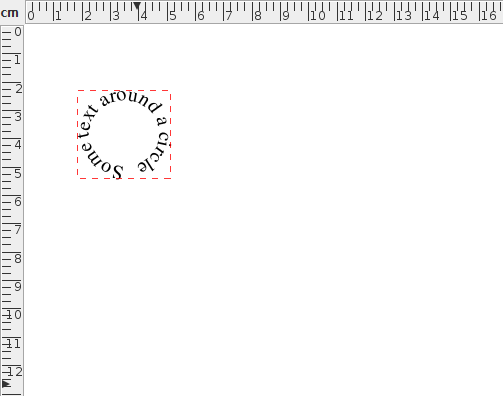(b)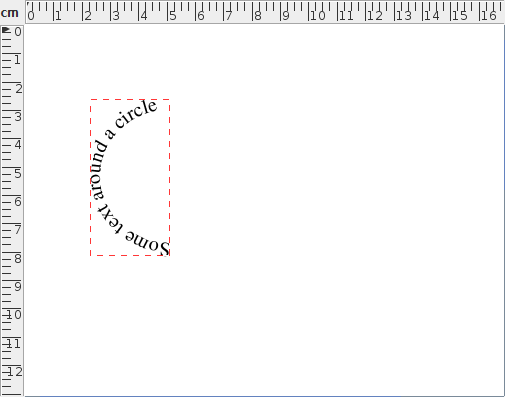(c)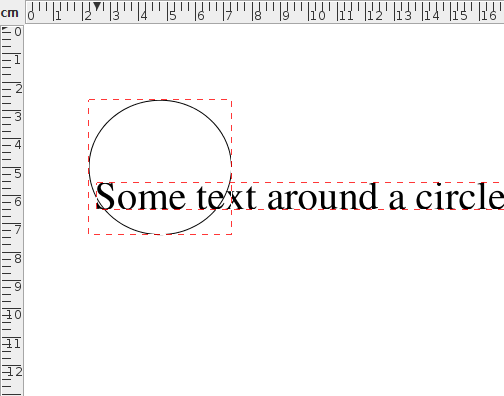(d)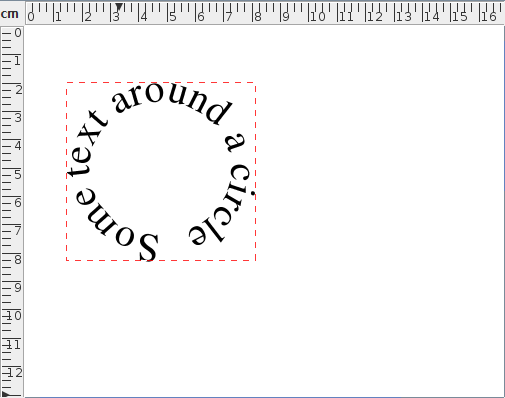(e)
Figure 8.22: Scaling a text-path: (a) original text area and path; (b) text area and path in (a) combined to form a text-path; (c) text-path in (b) scaled by a factor of 2; (d) text area and path in (a) scaled by a factor of 2; (e) scaled text area and path in (d) combined to form a text-path.

If you prefer to scale an object using the mouse, you first need to enable the hotspots. Then drag the bottom centre hotspot to scale vertically, the bottom right hotspot to scale in both directions or the middle right hotspot to scale horizontally. Note that even if you have more than one object selected, only the object whose hotspot you are dragging will be transformed.